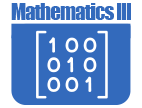# Math - Secondary Math III

IntroductionIN SECONDARY MATHEMATICS III students pull together and apply the accumulation of learning that they have from their previous courses, with content grouped into four critical areas, organized into units. They apply methods from probability and statistics to draw inferences and conclusions from data. Students expand their repertoire of functions to include polynomial, rational, and radical functions. They expand their study of right triangle trigonometry to include general triangles. And, finally, students bring together all of their experience with functions and geometry to create models and solve contextual problems. The Mathematical Practice Standards apply throughout each course and, together with the content standards, prescribe that students experience mathematics as a coherent, useful, and logical subject that makes use of their ability to make sense of problem situations.

CRITICAL AREA 1: Students see how the visual displays and summary statistics they learned in earlier grades relate to different types of data and to probability distributions. They identify different ways of collecting data - including sample surveys, experiments, and simulations—and the role that randomness and careful design play in the conclusions that can be drawn.

CRITICAL AREA 2: This area develops the structural similarities between the system of polynomials and the system of integers. Students draw on analogies between polynomial arithmetic and base-ten computation, focusing on properties of operations, particularly the distributive property. Students connect multiplication of polynomials with multiplication of multi-digit integers, and division of polynomials with long division of integers. Students identify zeros of polynomials and make connections between zeros of polynomials and solutions of polynomial equations. The unit culminates with the fundamental theorem of algebra. Rational numbers extend the arithmetic of integers by allowing division by all numbers except 0. Similarly, rational expressions extend the arithmetic of polynomials by allowing division by all polynomials except the zero polynomial. A central theme of this unit is that the arithmetic of rational expressions is governed by the same rules as the arithmetic of rational numbers.

CRITICAL AREA 3: Students develop the Laws of Sines and Cosines in order to find missing measures of general (not necessarily right) triangles. They are able to distinguish whether three given measures (angles or sides) define 0, 1, 2, or infinitely many triangles. This discussion of general triangles opens up the idea of trigonometry applied beyond the right triangle—that is, at least to obtuse angles. Students build on this idea to develop the notion of radian measure for angles and extend the domain of the trigonometric functions to all real numbers. They apply this knowledge to model simple periodic phenomena.

CRITICAL AREA 4: Students synthesize and generalize what they have learned about a variety of function families. They extend their work with exponential functions to include solving exponential equations with logarithms. They explore the effects of transformations on graphs of diverse functions, including functions arising in an application, in order to abstract the general principle that transformations on a graph always have the same effect regardless of the type of the underlying functions. They identify appropriate types of functions to model a situation, adjust parameters to improve the model, and compare models by analyzing the appropriateness of the fit and making judgments about the domain over which a model is a good fit. The description of modeling as “the process of choosing and using mathematics and statistics to analyze empirical situations, to understand them better, and to make decisions” is at the heart of this area. The narrative discussion and diagram of the modeling cycle should be considered when knowledge of functions, statistics, and geometry is applied in a modeling context.The Online Core Resource pages are a collaborative project between the Utah State Board of Education and the Utah Education Network. If you would like to recommend a high quality resource, contact Trish French (Elementary) or Lindsey Henderson (Secondary). If you find inaccuracies or broken links contact resources@uen.org.